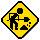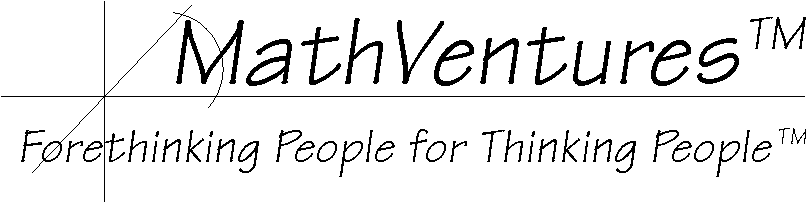Hard hat area, construction in progress# Logic  the Art of Reasoning

Mathematics   the Art of Studying Patterns Using Logic

# Subtraction Without Borrowing  Just Do It!Uri Geva, MathVentures, a Division of Ten Ninety

Document Version 1.20 (Just Do-It)
This work comes with absolutely no warranty!

# Its Simple and Easy

Subtracting without borrowing is very simple and easy. (It does not use either compliment arithmatic or negative numbers.) It can easily be adopted to be your everyday subtracting method. You can learn how to do it by simply following the two examples described below.
 A note for the mathematically advanced reader (If you do not think of yourself to be one, skip this note): There are other methods for performing subtraction without borrowing. Some involve the use of negative numbers and others use methods of complements, either 9-Complement or 10-Complement.  The technique described here has several advantages over these methods.
• This method is very simple.
• It requires no more than the mathematical knowledge of a second or third grader. No need to know special concepts as negative numbers and complements.
• Often students, who have to perform subtraction, intuitively take a short-cut  they round at least one of the numbers up or down to a number with which they feel more comfortable. This method is nothing more than a general and algorithmic description of this alternative approach.

Examples:
Editorial Note:The text below identifies the numbers involved in a subtraction      operation using the following names:
9 <== the minuend
 4 <== the subtrahend
5 <==the result
Notice: For best result viewing the following examples, maximize your browser's window to avoid line wrap.

 StepNo. Action Description The Numbers' Names Example 1 Example 2 1) Example 1. Ordinarily, borrowing is needed right away and in everycolumn except for the last, leftmost one. The Minuend ==> The Subtrahend ==> 87,352  49,684 Example 2. Ordinarily, borrowing is needed only starting with the third column from the right. The Minuend ==> The Subtrahend ==> 675,732   75,921 2) Change every digit except for the leftmost one of the Minuend to 9. The Minuend ==> 89,999 699,999 3) Subtract 1 from the leftmost digit of the minuend in Step #2. The Minuend ==> 79,999 599,999 4) Add 1 to the part of the original minuend that we changed to 9's in Step #2. This sum equals to... the difference between the original  minuend and the result of Step #3. The changed part of the Minuend ==> 1==> The Sum ==>   Original Minuend ==> The Minuend==> The Difference ==> 7,352 +    1 7,353   87,352  79,999 7,353 75,732 +      1 75,733 675,732  599,999 75,733 5) Remember this number Remembered Number ==> 7,353 75,733 6) Write the new subtraction, using the new minuend New Minuend ==> The Subtrahend ==> 79,999  49,684 599,999  75,921 7) Now the subtraction is simple. Do it in each column in any order you wish. New Minuend ==> The Subtrahend ==> Temp Result ==> 79,999  49,684 30,315 599,999  75,921 524,078 8) Add to the Temp Result of Step 7 the Remember Number  in Step 5. Remembered Number ==> Final Result ==> + 7,353 37,668 + 75,733 599,811
Thats it! If it seems complex, it is only because a written description must be explicit and detailed. When Demonstrating it to students in person, they get it right away. [We plan to post here an animated demonstration with graphic and voice to simulate such personal explanation.]. Practice it a few times and youll realize how simple and easy it is.The Trick If You Want To Understand How It Works

The basic idea is simple too.

Borrowing in necessary because one or more digits in the subtrahend are larger than the corresponding digit in the minuend. Only the leftmost digit of the minuend is larger than the corresponding digit in the subtrahend.

So what we do is this:

1. Replace all of the digit of the minuend, except this leftmost one, by 9 and reduce the leftmost one by 1. This guarantees us a temporary

2. minuend of which every digit is equals to or larger than the corresponding digit in the subtrahend.

3. Now subtraction is very simple.

4.
5. But we must add to the result the difference between the original minuend and the minuend that we used in the subtraction. Because we used only 9s in the temporary minuend, this difference equals to the number that is made from the digits that we changed plus 1. So even here we do not need to do any subtraction.
See, the summary of the procedure is quite simple.

Following this subtraction procedure you do

• one simple single-digit subtraction, one operation for each column of digits, and

Now that you've seen it, make your own exercises and practice. You'll master it in no time.